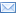# New Methods for the Perfect-Mirsky Conjecture and Private Quantum Channels

 Title: New Methods for the Perfect-Mirsky Conjecture and Private Quantum Channels Levick, Jeremy Department of Mathematics and Statistics Mathematics and Statistics Pereira, Rajesh The Perfect-Mirsky conjecture states that the set of all possible eigenvalues of an n by n doubly stochastic matrix is the union of certain specific $n-1$ regular polygons in the complex plane. It is known to be true for $n=1,2,3$ and recently a counterexample was found for $n=5$. We show that the conjecture is true for $n=4$. We also modify the original conjecture for $n>4$ in order to accommodate the n=5 counterexample, as well as provide evidence for our new conjecture. An important class of quantum channels are the Pauli channels; channels whose Kraus operators are all tensor products of Pauli matrices. A private quantum channel is a quantum channel that is non-injective for some set of inputs. Private quantum channels generalize the classical notion of the one-time pad to the quantum setting. PQCs are most useful when the set of inputs they privatize is isomorphic as an algebra to an algebra of qubits. We use the theory of conditional expectations to show that in certain cases, the set of algebras privatized by a channel is the set of algebras quasiorthogonal to the range of the channel. Quasiorthogonality is an idea from operator algebras that has begun to find applications elsewhere in quantum information. Using these ideas, we prove that conditional expectation Pauli channels with commuting Kraus operators can always privatize $\frac{\log(n)}{2}$ qubits, where $n$ is the number of Kraus operators. We also use ideas from Fourier analysis on finite groups to give a method for finding privatized algebras general Pauli channels. Using character theory, we extend this analysis beyond Pauli channels, and show how to find private algebras for qutrit and other channels. Finally, using ideas from Lie theory, we show a connection between quasiorthogonality and the Cartan decomposition of a Lie algebra, giving new methods for finding algebras privatized by a channel. We also use the $KAK$ decomposition of a Lie algebra to begin looking at ways to encode algebras so they are privatized. http://hdl.handle.net/10214/9128 2015-06 Attribution 2.5 Canada
﻿

## Files in this item

Files Size Format View Description
Levick_Jeremy_201509_PhD.pdf 632.9Kb PDF View/Open Thesis

### This item appears in the following Collection(s)Except where otherwise noted, this item's license is described as Attribution 2.5 Canada
The library is committed to ensuring that members of our user community with disabilities have equal access to our services and resources and that their dignity and independence is always respected. If you encounter a barrier and/or need an alternate format, please fill out our Library Print and Multimedia Alternate-Format Request Form. Contact us if you’d like to provide feedback: lib.a11y@uoguelph.ca